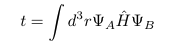# Outputting the hopping parameters (i.e. elements of the Hamiltonian)?

Hi,

is it possible to output the full Hamiltonian Matrix during a calculation? I ask because I want to get access to hopping parameters of my molecule and those are the elements of the Hamiltonian (see image).

Also, can I choose what orbitals to run a calculation with? For instance, can I choose to only run the s-orbitals available in my molecule for that?Can I ask what your background is in and what kinds of systems you’re interested in? It sounds like you’re interested in toy systems and are not a chemist by training.

I have a physics background but one of my projects deals with a peptide where I need to know the Hamiltonian of my system for a given set of orbitals. But before I get to that I want to know how to get the Hamiltonian for the simplest case. And the simplest case for me would be the Hubbard chain.

I don’t know what you mean by “know the Hamiltonian of my system for a given set of orbitals.” For any set of orthonormal orbitals, the molecular Hamiltonian is given as a linear combination of second quantized operators, with expansion coefficients given by one and two-electron integrals. Are you asking how you can get a program to compute the integrals for you? Are you trying to learn how to compute the integrals “from scratch”?

Psi is designed for molecular systems and does not support a Hubbard chain.

The “only s-orbital” approximation is not even qualitatively correct for most atoms. For hydrogen atoms, it’s qualitatively correct. Choose a STO-3G or STO-6G basis set, which is the bare minimum.

Forming the Hamiltonian matrix for a molecule is prohibitively expensive and is only ever done for pedagogical purposes. See here for an example.

The s orbital was referring to H2. What are the inputs for that case for instance?

This sounds like a question of basic Psi4 syntax. If you want to learn how to write an input file to compute the energy of H2 in the STO-3G basis set, please consult the many sample inputs we have available and read the manual.

If you still have a question after that, ask again.

I did the H2 example for the STO-3G basis. Reading through the manual I can see that what I want is probably given by the keyword HEFF_PRINT. But how do I set it to true? I did ‘set psimrcc HEFF_PRINT TRUE’ but that didn’t output me the Hamiltonian. Any ideas?

EDIT: I think I got it. Setting
Escf, scf_wfn = energy(‘SCF’, molecule=h2o, return_wfn=True)
scf_wfn.H().print_out()

I managed to print out the one-electron Hamiltonian.

`set psimrcc heff_print true` will only affect the PSIMRCC module, which I assure you is not what you want.

`scf_wfn.H()` is what quantum chemists call the core Hamiltonian. It is the matrix representation of the one-electron part of the Hamiltonian acting on the one-electron space. It does not represent the two-electron part of the Hamiltonian or wavefunctions of more than two electrons.

If you really want the Hamiltonian, see the link I included in my previous post.

And for the record, I still don’t know what you actually want. Do you want numerical values for matrix elements of the Hamiltonian between two-electron states of H2? Do you want formulas for those numerical values expressed in terms of integrals? Do you want formulas for the integrals?

I want the numerical values for matrix elements of the Hamiltonian between two-electron states.

Then yes, go look at the FCI pilot implementation I linked earlier.

You’ll need to change the molecule from H2O to H2, but that should be straightforward.

This topic was automatically closed 60 days after the last reply. New replies are no longer allowed.